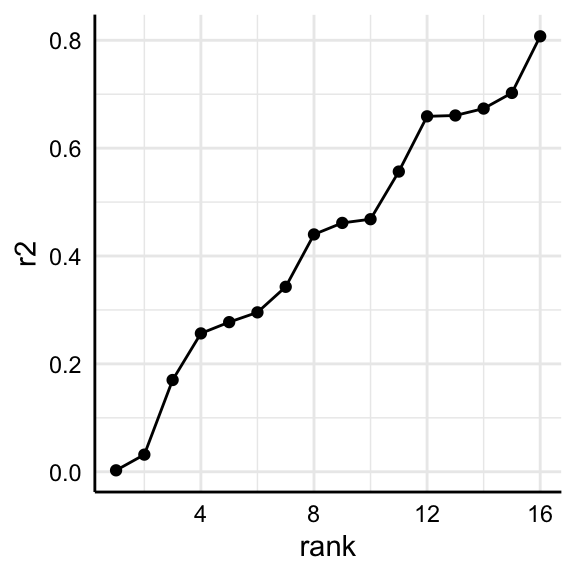# Investigating selected specifications

Per default, run_specs() summarizes individual specifications by using broom::tidy(). For most cases, this provides “good-enough” summaries of the relationship of interest. Sometimes, however, it might be useful to investigate specific models in more detail or to investigate a specific parameter that is not provided by broom::tidy() (e.g., r-square, AIC). This vignette shows how to access individual models.

## 1. Run specification curve analysis

If we want to investigate individual models and particularly all aspects of that model, we need to use run_specs() with the additional argument keep.results = TRUE.

library(specr)
library(dplyr)
library(purrr)
library(ggplot2)
library(performance)

# Run specification curve analysis
results <- run_specs(df = example_data,
y = c("y1", "y2"),
x = c("x1", "x2"),
model = c("lm"),
controls = c("c1", "c2"),
subsets = list(group1 = unique(example_data$group1), group2 = unique(example_data$group2)),
keep.results = TRUE)

## 2. Identify model(s) of interest

For this example, we are going to look at two specific models (same independent variables, all controls, all participants, but different dependent variables).

(y_models <- results %>%
filter(x == "x1",
controls == "c1 + c2",
subsets == "all"))
#> # A tibble: 2 x 13
#>   x     y     model controls res   estimate std.error statistic  p.value
#>   <chr> <chr> <chr> <chr>    <lis>    <dbl>     <dbl>     <dbl>    <dbl>
#> 1 x1    y1    lm    c1 + c2  <lm>    6.17       0.412    15.0   4.70e-42
#> 2 x1    y2    lm    c1 + c2  <lm>   -0.0545     0.267    -0.204 8.38e- 1
#> # … with 4 more variables: conf.low <dbl>, conf.high <dbl>, obs <int>,
#> #   subsets <chr>

As you can see, the resulting tibble includes an additional column called “res”. This column includes the entire “model object” and we can use it to further investigate each model.

## 3. Investigate models

For example, we can now easily get a full summary of the models and compare individual coefficients and statistics.

y_models %>%
pull(res) %>%
map(summary) %>%
map(coef)
#> []
#>               Estimate Std. Error   t value     Pr(>|t|)
#> (Intercept) -13.155786  1.2877892 -10.21579 2.305561e-22
#> x1            6.167539  0.4121184  14.96545 4.697951e-42
#> c1            3.305519  0.2120368  15.58937 7.001986e-45
#> c2            5.069924  0.2071211  24.47807 2.353281e-87
#>
#> []
#>               Estimate Std. Error    t value     Pr(>|t|)
#> (Intercept) -3.4181335  0.8345588 -4.0957372 4.913869e-05
#> x1          -0.0545206  0.2670756 -0.2041392 8.383285e-01
#> c1           3.3246890  0.1374116 24.1951149 5.457463e-86
#> c2           2.3954736  0.1342259 17.8465776 2.162445e-55

Or we could get r-squared values for both models.

y_models %>%
pull(res) %>%
map(r2)       # r2 is include in the package "performance"
#> []
#> # R2 for Linear Regression
#>
#>        R2: 0.702
#>
#> []
#> # R2 for Linear Regression
#>
#>        R2: 0.659
#>   adj. R2: 0.657

## 4. Some more examples

This way, we can analyze or compare such statistics across several models.

r2_results <- results %>%
filter(subsets == "all") %>%
mutate(r2 = map(res, r2),
r2 = map_dbl(r2, 1)) %>%
arrange(r2)

r2_results %>%
select(x:controls, r2)
#> # A tibble: 16 x 5
#>    x     y     model controls           r2
#>    <chr> <chr> <chr> <chr>           <dbl>
#>  1 x1    y2    lm    no covariates 0.00263
#>  2 x2    y2    lm    no covariates 0.0317
#>  3 x1    y1    lm    no covariates 0.170
#>  4 x1    y2    lm    c2            0.257
#>  5 x2    y2    lm    c2            0.277
#>  6 x2    y1    lm    no covariates 0.296
#>  7 x1    y1    lm    c1            0.343
#>  8 x1    y2    lm    c1            0.440
#>  9 x2    y2    lm    c1            0.461
#> 10 x2    y1    lm    c1            0.468
#> 11 x1    y1    lm    c2            0.557
#> 12 x1    y2    lm    c1 + c2       0.659
#> 13 x2    y1    lm    c2            0.661
#> 14 x2    y2    lm    c1 + c2       0.673
#> 15 x1    y1    lm    c1 + c2       0.702
#> 16 x2    y1    lm    c1 + c2       0.807

And we can plot comparisons.

r2_results %>%
arrange(r2) %>%
mutate(rank = 1:n()) %>%
ggplot(aes(x = rank,
y = r2)) +
geom_point() +
geom_line() +
theme_minimal() +
theme(strip.text = element_blank(),
axis.line = element_line("black", size = .5),
axis.text = element_text(colour = "black"))